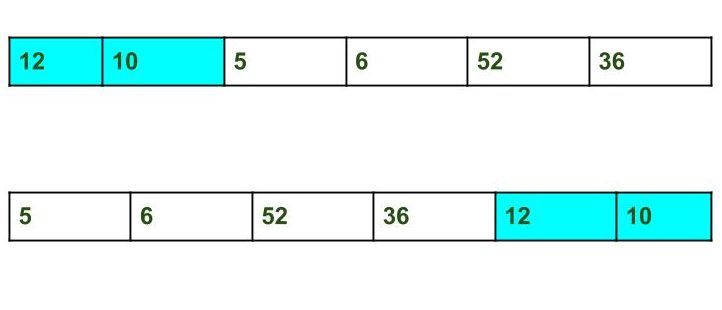GFG App
Open AppBrowser
Continue

# C++ Program to Split the array and add the first part to the end | Set 2

Given an array and split it from a specified position, and move the first part of array add to the end.Examples:

```Input : arr[] = {12, 10, 5, 6, 52, 36}
k = 2
Output : arr[] = {5, 6, 52, 36, 12, 10}
Explanation : Split from index 2 and first
part {12, 10} add to the end .

Input : arr[] = {3, 1, 2}
k = 1
Output : arr[] = {1, 2, 3}
Explanation : Split from index 1 and first

A O(n*k) solution is discussed here
This problem can be solved in O(n) time using the reversal algorithm discussed below,
1. Reverse array from 0 to n – 1 (where n is size of the array).
2. Reverse array from 0 to n – k – 1.
3. Reverse array from n – k to n – 1.

## C++

 `// C++ program to Split the array and ` `// add the first part to the end ` `#include ` ` `  `using` `namespace` `std; ` ` `  `/* Function to reverse arr[] from index start to end*/` `void` `rvereseArray(``int` `arr[], ``int` `start, ``int` `end) ` `{ ` `    ``while` `(start < end) { ` `        ``int` `temp = arr[start]; ` `        ``arr[start] = arr[end]; ` `        ``arr[end] = temp; ` `        ``start++; ` `        ``end--; ` `    ``} ` `} ` ` `  `// Function to print an array ` `void` `printArray(``int` `arr[], ``int` `size) ` `{ ` `    ``for` `(``int` `i = 0; i < size; i++) ` `        ``cout << arr[i] << ``" "``; ` `} ` ` `  `/* Function to left rotate arr[] of size n by k */` `void` `splitArr(``int` `arr[], ``int` `k, ``int` `n) ` `{ ` `    ``rvereseArray(arr, 0, n - 1); ` `    ``rvereseArray(arr, 0, n - k - 1); ` `    ``rvereseArray(arr, n - k, n - 1); ` `} ` ` `  `/* Driver program to test above functions */` `int` `main() ` `{ ` `    ``int` `arr[] = { 12, 10, 5, 6, 52, 36 }; ` `    ``int` `n = ``sizeof``(arr) / ``sizeof``(arr); ` `    ``int` `k = 2; ` ` `  `    ``// Function calling ` `    ``splitArr(arr, k, n); ` `    ``printArray(arr, n); ` ` `  `    ``return` `0; ` `} `

Output:

`5 6 52 36 12 10 `

Please refer complete article on Split the array and add the first part to the end | Set 2 for more details!

My Personal Notes arrow_drop_up